## Pages

### Seven segment based alarm clock using 8051 microcontroller (AT89C51)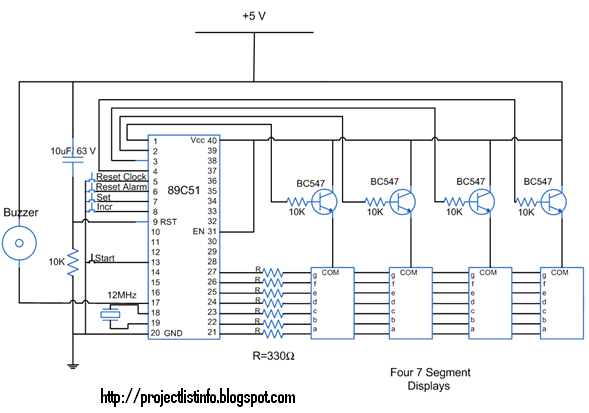An alarm clock is a clock that indicates a pre-set time by producing sound at that time. This functionality of digital clock is used to awaken people or remind them of something. This circuit is an extension to the digital clock with time setting option.  Here an extra switch is provided to set the alarm. While the alarm is set, the clock time does not stop and runs in the background. The project is build around the 8051 microcontroller (AT89C51)
To provide various options in the digital clock, following switches are used:
Switch 1 (S1)   :           Reset               (to initiate the time set option)
Switch 2 (S2)   :           Select              (to select the segment where value is to be changed)
Switch 3 (S3)   :           Increment        (to increase the value at selected segment)
Switch 4 (S4)   :           Start                (to start the clock with the set time options)
Switch 5 (S5)   :           Alarm              (to initiate the alarm set option)

The option to set alarm is provided by providing an extra switch S5, with the microcontoller At89C51, which is made active low. Whenever S5 is pressed, the time display goes off and only 1st segment is activated. But the clock time still runs in the background. In this mode, a segment can be selected in cyclic order by pressing S2. After selecting the desired segment, its value can be changed by using S3. Once the digits and hence the alarm is set, S4 is pressed to start the clock again. As soon as the set alarm time is reached, a buzzer gets signal from the microcontroller thus producing sound.

The seven segments are interfaced to port P2 of the microcontroller AT89C51 through its data pins (a - h). The enable pins are connected to pins 1-4 of port P1 (P1^0 – P1^3). The switches S1-S5 and buzzer are connected as following:
S1         :           P1^4                                         S4         :           P3^3
S2         :           P1^6                                         S5         :           P1^5
S3         :           P1^7                                         Buzzer :           P3^7

The buzzer is connected to the output pin of microcontroller at the negative end and to Vcc at the positive end.
C Code:
// Program to make a digital clock with time and alarm setting features

#include<reg51.h>
sbit dig_ctrl_4=P1^0;  //Declare the control pins for the seven segments
sbit dig_ctrl_3=P1^1;
sbit dig_ctrl_2=P1^2;
sbit dig_ctrl_1=P1^3;
sbit buzzer=P3^7;
sbit resetalarm=P1^5;  //Reset alarm pin to reset the alarm.
sbit resetclock=P1^4;  //Reset clock pin to reset the clock.
sbit start=P3^3;  // Start pin for starting the clock after time setting
sbit incr=P1^7;  // Increment pin to increase the digits during time setting.
sbit set=P1^6;  // Set pin to set the time.
int sel_seg_to_incr=0;
int ret_seg_to_incre=0;
int recnt_incr_seg;
int begin;
unsigned char dig_disp=0;
int min2=0;
int min1=0;
int sec2=0;
int sec1=0;
int minalarm2=0;
int minalarm1=0;
int secalarm2=0;
int secalarm1=0;
int alarmmin2=0;
int alarmmin1=0;
int alarmsec2=0;
int alarmsec1=0;
int keepmin2=0;
int keepmin1=0;
int keepsec2=0;
int keepsec1=0;
int loop=0;
int mode;
char dig_val={0x40,0xF9,0x24,0x30,0x19,0x12,0x02,0xF8,0x00,0x10};  // Hex values corresponding to digits 0 to 9

void delay(int time)  //Function to provide time delay.
{
int i,j;
for(i=0;i<time;i++)
for(j=0;j<1275;j++);
}

int setfn()  // Function to select miniute and seconds digit set time.
{
while(set==0)
{
switch(recnt_incr_seg)
{

case 1:
if(set==0)  //Select the min2 digit
{
dig_ctrl_4=1;
dig_ctrl_3=0;
dig_ctrl_2=0;
dig_ctrl_1=0;
recnt_incr_seg=1;
ret_seg_to_incre=1;
P2=dig_val[minalarm2];
delay(100);
}

case 2:
if(set==0)  //Select the min1 digit
{
dig_ctrl_4=0;
dig_ctrl_3=1;
dig_ctrl_2=0;
dig_ctrl_1=0;
recnt_incr_seg=2;
ret_seg_to_incre=2;
P2=dig_val[minalarm1];
delay(100);
}

case 3:
if(set==0)  //Select the sec 2 digit
{
dig_ctrl_4=0;
dig_ctrl_3=0;
dig_ctrl_2=1;
dig_ctrl_1=0;
recnt_incr_seg=3;
ret_seg_to_incre=3;
P2=dig_val[secalarm2];
delay(100);
}

case 4:
if(set==0)  //Select the sec1 digit
{
recnt_incr_seg=1;
dig_ctrl_4=0;
dig_ctrl_3=0;
dig_ctrl_2=0;
dig_ctrl_1=1;
ret_seg_to_incre=4;
P2=dig_val[secalarm1];
delay(100);
recnt_incr_seg=1;
}
}
}
return(ret_seg_to_incre);
}

void increase(int a)  //Function to set the minutes or seconds digit
{
while(incr==0)
{
switch(a)
{

case 1:  // Set the min2 digit.
P2=dig_val[minalarm2];
delay(100);
minalarm2++;
if(minalarm2==6)
minalarm2=0;
P2=dig_val[minalarm2];
delay(20);
break;

case 2:  //Set the min1 digit.
P2=dig_val[minalarm1];
delay(100);
minalarm1++;
if(minalarm1==10)
minalarm1=0;
P2=dig_val[minalarm1];
delay(20);
break;

case 3:  // Set the sec2 digit.
P2=dig_val[secalarm2];
delay(100);
secalarm2++;
if(secalarm2==6)
secalarm2=0;
P2=dig_val[secalarm2];
delay(20);
break;

case 4:  //Set the sec1 digit.
//recnt_incr_seg=4;
P2=dig_val[secalarm1];
delay(100);
secalarm1++;
if(secalarm1==10)
secalarm1=0;
P2=dig_val[secalarm1];
delay(20);
break;
}
}
}

void resetfn(mode)  // Function to bring the clock to reset or set mode.
{
begin=1;
dig_ctrl_4=1;  //Enable the min2 digit and disable others
dig_ctrl_3=0;
dig_ctrl_2=0;
dig_ctrl_1=0;
if(mode==0)  //Check if clock is in set alarm mode
{
IE=0x88;  //Disable Timer0 interrupt to stop the display of clock.
sel_seg_to_incr=1;
recnt_incr_seg=1;
P2=dig_val[keepmin2];
delay(100);
minalarm2=keepmin2;
minalarm1=keepmin1;
secalarm2=keepsec2;
secalarm1=keepsec1;
}
if(mode==1)  //Check if clock is in set clock mode
{
IE=0x80;  //Disable Timer0 interrupt to stop the clock.
minalarm2=min2;
minalarm1=min1;
secalarm2=sec2;
secalarm1=sec1;
sel_seg_to_incr=1;
recnt_incr_seg=1;
P2=dig_val[min2];
delay(100);
}
while(1)
{
if(start==0)  //Check if start pin is pressed
{
if(mode==0)
{
keepmin2=minalarm2;
keepmin1=minalarm1;
keepsec2=secalarm2;
keepsec1=secalarm1;
alarmmin2=minalarm2;
alarmmin1=minalarm1;
alarmsec2=secalarm2;
alarmsec1=secalarm1;
}
if(mode==1)
{
min2=minalarm2;
min1=minalarm1;
sec2=secalarm2;
sec1=secalarm1;
}
TMOD=0x11;  //Reset the timer0
TL0=0xf6;
TH0=0xFf;
IE=0x8A;  //Enabling Timer0 interrupt to start the display of clock
TR0=1;
break;
}
if(set==0)  //Check if set pin is pressed
sel_seg_to_incr=setfn();
if(incr==0)  //Check if incr pin is pressed
increase(sel_seg_to_incr);
}
}

void display() interrupt 1  // Function to display the digits on seven segment using the concept of seven segment multiplexing.
{
TH0=0xf6;
P2=0xFF;
dig_ctrl_1 = dig_ctrl_3 = dig_ctrl_2 = dig_ctrl_4 = 0;
dig_disp++;
dig_disp=dig_disp%4;
switch(dig_disp)
{
case 0:
P2=dig_val[sec1];
dig_ctrl_1 = 1;
break;

case 1:
P2= dig_val[sec2];
dig_ctrl_2 = 1;
break;

case 2:
P2= dig_val[min1];
dig_ctrl_3 = 1;
break;

case 3:
P2= dig_val[min2];
dig_ctrl_4 = 1;
break;
}
}

void moveclock() interrupt 3  // Function to increment clock digits
{
loop++;
if(loop==20)
{
sec1++;
if(sec1==10)
{
sec1=0;
sec2++;
if(sec2==6)
{
sec1=0;
sec2=0;
min1++;
if(min1==10)
{
sec1=0;
sec2=0;
min1=0;
min2++;
if(min2==6)
{
sec1=0;
sec2=0;
min1=0;
min2=0;
}
}
}
}
loop=0;
}
}

void main()
{
mode=0;
set=1;  //Initialize set, reset, start and incr pins as input
resetalarm=1;
resetclock=1;
start=1;
incr=1;
begin=0;
TMOD=0x11;  //Intialize Timer 0
TH0=0xFf;
IE=0x8A;  //Enable Timer0 interrupt
TR0=1;  //Start Timer0
TL1=0xFD;
TH1=0x4B;
TR1=1;  // Start Timer1
while(1)
{
h
if(resetalarm==0)  //Check if reset alarm pin is pressed
{
resetfn(0);
}
if(resetclock==0)//Check if reset clock pin is pressed
{
resetfn(1);
}
if(min2==alarmmin2&&min1==alarmmin1&&sec2==alarmsec2&&sec1==alarmsec1&&begin==1)// Check for Alarm condition
{
buzzer=0;
delay(500);
buzzer=1;
}
}

}

1.oloyetunde@gmail.com

2.orlando7817@gmail.com

3.orlando7817@gmail.com

4.bluecrystal_sapphire@hotmail.com

5.bluecrystal_sapphire@hotmail.com

6.help me with the code

7.bkash73@yahoo.com

8.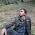kandelhem049@gmail.com
please design a circuit of seven segment based time and date display using DS1307 or DS12887 with microcontroller AT89C51

9.kandelhem049@gmail.com
please design a circuit of seven segment based time and date display using DS1307 or DS12887 with microcontroller AT89C51

10.macasayaryan@gmail.com

11.macasayaryan@yahoo.com

12.ngk14@yahoo.in plz send d code

13.I would really appreciate it if you could give me the C code
My email address is
archerf97@gmail.com

14.khansalman@engineer.com
plz send...

15.saiganesh1919@gmail.com
please send me the circuit n coding for making seven segment digital clock showing just hour and mintute with time setting option using microcontroller AT89S51

16.saiganesh1919@gmail.com
please send me the circuit n code for making a seven segment clock using microcontroller AT89S51 showing only hour n minute with time setting option

17.brianngiri@gmail.com

18.ssaumyamehta14@gmail.com
plz send me the circuit diagram and c code for seven segment clock using micro controller AT89c51

19.ssaumyamehta14@gmail.com
plz send me the circuit diagram and c code for making a seven segment clock using micro controller AT89c51

20.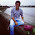bestintheworldrohit@y7mail.com

21.Please send me the code to my school email kramer@email.itt-tech.edu

22.Hi,
would I be able to set up another alarm by attaching a switch and the counters to the pins 39-35? I'm not yet completely sure what these pins are. I'm kind of hoping I can use them as input output ports like the other pins.

23.anplateef@yahoo.com
plz send me the circuit diagram and c code for making a seven segment clock using micro controller AT89c51

24.bharatmanchanda312@gmail.com plz send me tha project reprt also

25.Good project. Simple & practice. please send me source code and circuit diagram for this project. Thanks.

26.Good project. Simple & practice. Please send me source code and circuit diagram for this project. Thanks.

27.Good project. Simple & practice. Please send me source code and circuit diagram. Thanks.

28.Good project...! simple and practice. Please send me source code and circuit diagram

29.x_warrior5259@live.com

please send me the circuit diagram and c code for making a *LCD clock by using micro controller AT89c51

30.donthackitagain@gmail.com

31.shrikant_bhoyar@mail.com

32.pls send me the code
y.j.mahajan1312@gmail.com

33.bindass.sukanya@gmail.com

34.bindass.sukanya@gmail.com

35.please send me the code in c my email id is deep0177@gmail.com

36.please send me the code my email id is deep0177@gmail.com

37.jgarcia349@email.itt-tech.edu

plz send me the circuit diagram and c code for making a seven segment clock using micro controller AT89c51

38.Jgarcia349@email.itt-tech.edu
plz send me the circuit diagram and c code for making a seven segment clock using micro controller AT89c51

39.40.zmotion1@gmail.com

41.plz send me code.

42.arekrishnateja@gmail.com

43.arekrishnateja@gmail.com

44.pls send me the c code and if possible pls provide me the details of how to operate multiple pushbuttons as follows. one for start/ stop , second button for digitselection and third for increment/decrement
seeni.rinku@gmail.com

45.i need code in assembler language

shanmukhasrk@yahoo.com

46.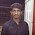I would really appreciate it if you could give me the C code
My email address is raki.p.nttf@gmail.com

47.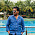Please send me d c code

48.plz send me assembly language code for this
maha.malik@yahoo.com

49.luchiant@gmail.com
Please send me the c code and a larger schematic.

50.umairali766@gmail.com

51.plz send me the c code or hex code at vekariyaarin@gmail.com

52.kanna_muthu@ymail.com
thnkz

53.Please Send the c code to

thegoldendart@gmail.com

54.ioan_S2000@yahoo.com

IF you any query about any project related than write your comment in comment box